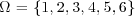2.4 EVENTS AND FIELDS

The sample space contains all possible outcomes of an experiment; these outcomes are the elements (points) of Ω. However, in most applications we are not usually interested in single outcomes, but in subsets of Ω called events.

Definition: Event Event E of an experiment is a subset of the sample space Ω whose elements share a common feature.

Example 2.14. The experiment where two coins are tossed simultaneously has the four outcomes shown in Figure 2.3 which are the four elements of Ω. Consider the event of at least one H given by E = {HH, HT, TH}. Thus, E is not a specific outcome, but is instead a feature of three of the four possible outcomes. Obviously, E is a subset of Ω.

FIGURE 2.3 Sample space for two coins tossed simultaneously showing two nonelementary events.Example 2.15. For the experiment of tossing a single die, the sample space is. Subset E = {1, 3, 5} is the event that the die shows an odd value.

Example 2.16. In an experiment where two dice are tossed, the event that they show the same value is the subset A = {{1, 1}, {2, 2}, {3, 3}, {4, 4}, {5, 5}, {6, 6}}.

Example 2.17. For, A = [0, 1/2] and are examples of events.

Note that an element ...

Get Probability, Random Variables, and Random Processes: Theory and Signal Processing Applications now with the O’Reilly learning platform.

O’Reilly members experience books, live events, courses curated by job role, and more from O’Reilly and nearly 200 top publishers.